# PMSM FOC - problem with higher velocity

#### Krzysztof Bieda

Joined Jan 5, 2016
46
Hello everyone,

I am developing a controller and I have implemented a FOC algorithm.
Motor is 24V, 10 magnet poles with build in 12bit magnetic encoder.
PWM: 20kHz. Contol interrupt: 2kHz.
On output I puted PWM SVM.

Everything seems to be fine in control up to 75rpm. I made a software to monitor it from PC: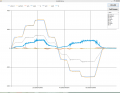Above that level I am getting unstable behaviour. I have tried to tune PI controlers but I did not find enything.
When it fails - it looks like that: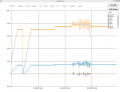In FOC algorithm Vd is not limited, but Vq is that sqrt(Vd*Vd + Vq*Vq ) =0.95 max

The problem is that I am not able to spin more than 75rpm, when nominal motors velocity is 190rpm.

I have observed currents: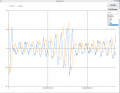I see that the problem is with d line: Id and Vd.
I have set Id on zero level.

Does anyone know how to solve that?

Best regards,
Christoph

#### sailorjoe

Joined Jun 4, 2013
364
Krzysztof, it's difficult to interpret your pictures without knowing where in your circuit the values are being measured. However, on your last picture, it looks like there is a large phase difference between the speed you want and the speed you get. That could begin to explain why you can't go faster. Somewhere in your system there is perhaps a large delay that is causing the phase shift.

Can you post a schematic with measurement points, please?

#### tsan

Joined Sep 6, 2014
138
What the signals are? For example setId. If i just guess it is flux related current on rotor coordinate but it can be what ever. Is x axis on the chart time in seconds? Please scale signal amplitudes to percent of for example nominal value. For example if magnetizing current is shown as number 45 it means nothing. But if it is shown as 3% of motor nominal current it is informative.

What is maximum output voltage of your inverter? I assume it is at least 24 V for 24 V motor but I don't know. If operation below 75 rpm is tested to be good then the problem can be lack of voltage. Back emf overcomes inverter output voltage and oscillation starts. Back emf at 75 rpm on 190 rpm motor should not be a problem. Do you have a signal of output voltage amplitude? It would be good to compare it to maximum output voltage (sometimes I have seen a signal called modulation index). Reason for oscillation can also be noload situation. Can you get some load to the motor?

Is it working correctly below 75 rpm? Please can you describe your FOC a little bit. If there is some flux related current controller (is this id and vd you wrote) what is the output? I guess it should be small on pmsm. Also torque current should be low on no load situation. How it behaves if you put some load to the motor?

#### Krzysztof Bieda

Joined Jan 5, 2016
46
Tsan:

Signals are like in theory for standard FOC.
Yes, X axis is scaled to time in seconds ( I am sending data from Microcontroller with known rate).

About the scalling, it is more compex because I only know that 40A is nominal for this motor.
Current sensors are ACS758 (200A), in reference controller that we normally used for this motor thare was 150A for this motor measured.

24V is max and also reference controller works with that voltage on this motor.

I have noload now for development, but also my reference controller spins that motor up to 120rpm without load.

Yes, it works correctly bellow 75rpm but I also see that if I put a load on 75rpm then it malfunctions, but on 60rpm then it behaves allright.

Yes, I see that Vd (flux) and Vq (torque) are quite big as there is noload.
I do not know why.

#### Krzysztof Bieda

Joined Jan 5, 2016
46
I am also not sure about control frequency I have.

20kHz is the PWM frequency.
Every 500us ( 2kHz ) I run control loop and then the output value is set.

For 120rpm, 10 magnet pairs current wave sin frequency should be 200Hz.

Maybe the problem is that I must apply more frequent control loop.

#### tsan

Joined Sep 6, 2014
138
I am also not sure about control frequency I have.

20kHz is the PWM frequency.
Every 500us ( 2kHz ) I run control loop and then the output value is set.

For 120rpm, 10 magnet pairs current wave sin frequency should be 200Hz.

Maybe the problem is that I must apply more frequent control loop.
Can you make your control loop faster to see if it makes operation better? You can make it also slower but 2 kHz is already on the slow side.

Is the motor 10 magnet poles like on mentioned on your first post or 10 polepairs? Anyway I get much smaller sin frequency. Even with 10 polepairs I get nominal frequency fn = (190 * 10)/ 60 = 31,6 Hz. 120 rpm would be 20 Hz. Is your 200 Hz typo and you meant 20 Hz?

#### tsan

Joined Sep 6, 2014
138
About the scalling, it is more compex because I only know that 40A is nominal for this motor.
Current sensors are ACS758 (200A), in reference controller that we normally used for this motor thare was 150A for this motor measured.
It is possible to correct current scaling error on PI controllers but it is better to know that scaling is correct. It would be good to supply current through ACS758 and measure what comes from ADC. Also check what comes from ADC without current to see if there is a big offset. Current can be DC current and it would be good to get at least 10 A. If measuring with DC measure both directions.
I have noload now for development, but also my reference controller spins that motor up to 120rpm without load.
Motor nominal speed is 190 rpm. Can it run 190 rpm?
Yes, I see that Vd (flux) and Vq (torque) are quite big as there is noload.
I do not know why.
I think it is ok that amplitude of Vq is in proportion to speed. Even with FOC I assume in practise V/F (motor stator voltage/stator voltage frequency) is close to V/F line. Stator voltage (let's say Vref to modulator) is Vref = sqrt(Vd^2 + Vq^2). Flux is pretty much handled by permanent magnet so Vq should contribute almost all the Vref. Now Vd and Vq are close the same. What can cause that? Could it be problem with encoder measurement, angle calculation or Park transformation. Btw, this is an example where it would be nice that Vd and Vq are scaled to some units. For example at 60 rpm we expect Vq to be about 60/195 *100=30% of nominal motor voltage If I understand this about correct.

For troubleshooting I would first check current scaling and make control signals scaling to some known units. How you know there is no overflow or PI controller saturation (if antiwindup is programmed) etc. if scalings are unknown. Or current scaling is so that there is almost only noise and no real current signal. I guess there is filtering somewhere for current signals. Especially if it is on software you can try to reduce it. Check DC voltage that it does not oscillate.

Then one could make a test run with reference system for example at 60 rpm and measure motor current and motor voltage. It can be compared to new system.

#### tsan

Joined Sep 6, 2014
138
24V is max and also reference controller works with that voltage on this motor.

I have noload now for development, but also my reference controller spins that motor up to 120rpm without load.
Is the 24V motor rating AC voltage phase to phase? Industrial low voltage motors (400 V and higher) are. I somehow mentally connected 24 V to related to truck battery which is DC. If the motor is 24 V AC phase to phase then 120 rpm out of 190 rpm nominal is about maximum speed you get from 24V DC without resorting to field weakening. Still there is available voltage at 75 rpm so it does not explain why problems start at 75 rpm.

#### Krzysztof Bieda

Joined Jan 5, 2016
46
My control loop calculation lasts 270us. Many float calculations. I wanted to be sure that I am not loosing data on integer up or down limit.
I can make it 300us the fasetest. ( now 500us).

That motor has 20 poles – 10 magnet pole pairs.

I have checked what is on ACS758 and I have zero Level on 1990.
ADC is 4096, and I have ref voltage 3V that I reference too on ADC.

I am not sure If I have a proper sensor, because it is +/- 200A.
I know that in reference controller the max current ( startup ) is 150A, and nominal for work is 40A.

190rpm is nominal, but as I see on reference ( bought) controller – 120 rpm is max for 24V. The controller that I build have to be the same in operation like ref – so I assume 120 as max.

V ref is 2999, so I must Vref = sqrt(Vd^2 + Vq^2)

I do not scale current readings – 1 A is 10 in digits. About filtering – I have not applied any.
I only have low pass filter on input - 15kHz.

That is my PI function. Maybe here I have a mistake:

signed int16 PIregulator(PIParam* REG, signed int16 setValue, signed int16 readValue)
{
//Normalize input values to be <-1,1>
REG->setValue=setValue;
//Normalized value of error
REG->In=error*(REG->ScaleIN);
//Integral Part
float32 integral_new= REG->IntegralOld + (REG->In) * (REG->dt);
//Antiwindup for integral
signed int16 max=(REG->ScaleOut);
// if (REG->Out > max || REG->Out <-(max) ) integral_new = REG->IntegralOld;
//New output calculation
float32 new_out=(REG->Kp)*(REG->In) +(REG->Kp)*(REG->Ki)*(integral_new);
//Output value Scalling
REG->OutNotScaled = new_out;
new_out = new_out*(float)REG->ScaleOut;
signed int16 out = (signed int16) new_out;
REG->OutNotLimited = out;
//Output saturation
if(out >= max) out= max;
if(out <= -max) out= -max;
//Values update
REG->IntegralOld=integral_new;
REG->errorOld=REG->In;
REG->Out=out;
return out;
}
Motor is 24V DC. BLDC from Micromotor: http://www.micromotor.ch/en/brushless-drives/bl-wheel-hub-motor.html

#### Krzysztof Bieda

Joined Jan 5, 2016
46
Above is explanation, and now I fill explain what I have noticed and where the problem probably is.
Before I got 10 magnet pair motor, I have used 3 magnet pair (cheaper) motor for tests.
I have developed there a code and later switched to 10 magnet pair.

On 3 pole - I have observed: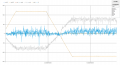and as you see. Here I had 120 rpm ( in my code I multiply it by 100 to have better resolution).

On that picture I see that for the same PI structure ( different KP and KI values) and the same
ClarkePark and Inverse ClarkePark caluclations - I had good results.

Something is wrong with measured Id and Iq on new motor, because as I see on picture above - Vd is always about average 100,
where on new motor I have it changing and different.

I spotted that Vd value depends on angle offset ( difference between measures sensor angle and the angle that I use for FOC calculation).

Do you know how to find optinal one for coil position?

#### Krzysztof Bieda

Joined Jan 5, 2016
46
Sorry, I analized it once more and it is 200us

#### tsan

Joined Sep 6, 2014
138
My control loop calculation lasts 270us. Many float calculations. I wanted to be sure that I am not loosing data on integer up or down limit.
I can make it 300us the fasetest. ( now 500us).
If tested with 300us one could see if it has any effect.

I have checked what is on ACS758 and I have zero Level on 1990.
ADC is 4096, and I have ref voltage 3V that I reference too on ADC.

I am not sure If I have a proper sensor, because it is +/- 200A.
I know that in reference controller the max current ( startup ) is 150A, and nominal for work is 40A.
So you use 1990 as offset for current reading. If 150 A should be possible then 200A range is ok.

V ref is 2999, so I must Vref = sqrt(Vd^2 + Vq^2)
This sentence I don't quite understand. I just mean that amplitude of Vref=sqrt(Vd^2 + Vq^2).

That is my PI function. Maybe here I have a mistake:
On quick look I see extra use of Kp on integral part calculation.
//New output calculation
float32 new_out=(REG->Kp)*(REG->In) +(REG->Kp)*(REG->Ki)*(integral_new);
Integral part has it's separate gain, Ki. Kp is extra and should not be there.

Do you know that back emf of the motor is sinusoidal? The term BLDC is often used for machine that has trapezoidal back emf. That kind of machine can't be run with FOC. The term PMSM is often used for machine that has sinusoidal back emf and it is controlled with FOC. Rotate the motor by hand or something and measure back emf with oscilloscope or something that shows back emf voltage waveform. It has to be sinusoidal for FOC. At the same time you see amplitude of back emf. Perhaps 75 rpm already is near 24 V and your reference controlled utilize field weakening.

#### Krzysztof Bieda

Joined Jan 5, 2016
46
V ref is 2999, so I must Vref = sqrt(Vd^2 + Vq^2)
I meant that max Vref is 2999, is is max PWM value I can put to reqister ( CPU 120MHz, PWM 20KHz)

I do not know if back emf is sinusoidal, but looking on reference power signals I see that every of coil has continous PWM signal.
That means it is sinusoidal.
In trapezoidal - you change power branch every 60 electrical angle. I have that algorithm too and it looks different on power signals.
Or maybe you know trapezoidal way of control where all three branches are used.

I discovered something trivial and probably explaining everything. As for now - all my knowledge about that motor was taken from datasheet given me by my boss. It was not the motor I haveThat is on the back of motor: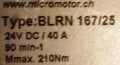So my 75rpm is close to nominal and probably field weakening is needed.

#### tsan

Joined Sep 6, 2014
138
Before I got 10 magnet pair motor, I have used 3 magnet pair (cheaper) motor for tests.
Is back emf sinusoidal both on 3 magnet pair motor and 10 magnet motor?

Something is wrong with measured Id and Iq on new motor, because as I see on picture above - Vd is always about average 100,
where on new motor I have it changing and different.
Is your reference controller from micromotor or something else? If it is FOC it is best to to look what it shows for Id, Iq etc. Btw, Micromotors Wheel Hub Motor BL167 noload speed is 190 rpm and voltage 24 V DC so you should be able to run 190 rpm with reference controller. Before further checking it has to be known that motor back emf is sinusoidal.

I spotted that Vd value depends on angle offset ( difference between measures sensor angle and the angle that I use for FOC calculation).

Do you know how to find optinal one for coil position?
I don't know but two ideas. If you have to find starting position perhaps it is possible to apply DC current to for example two phases so that rotor turns to known position (according to dc current) and start angle calculation from that. Or, make very small changes to angle offset while running (for example steady 50 rpm). Monitor current and voltage and make small changes so long that operation point is the same than with reference controller.

#### tsan

Joined Sep 6, 2014
138
I do not know if back emf is sinusoidal, but looking on reference power signals I see that every of coil has continous PWM signal.
That means it is sinusoidal.
But it is important to know that the motor back emf is sinusoidal (or trapezoidal) that correct control method is used.

In trapezoidal - you change power branch every 60 electrical angle. I have that algorithm too and it looks different on power signals.
Or maybe you know trapezoidal way of control where all three branches are used.
Measure the bemf waveform. If it is sinusoidal then there is some further troubleshooting/testing. If it is trapezoidal, test with your trapezoidal control code. To my knowledge trapezoidal is controlled so that current flows on two phases at any given time and the unused phase is used as position sensor.

I discovered something trivial and probably explaining everything. As for now - all my knowledge about that motor was taken from datasheet given me by my boss. It was not the motor I haveThat is on the back of motor:
View attachment 98299

So my 75rpm is close to nominal and probably field weakening is needed.
It does not explain big Vd before 75 rpm but could very well be the reason for problem at 75 rpm. Or perhaps on this motor Vd should be like that. What is Vd on reference controller?

#### tsan

Joined Sep 6, 2014
138
V ref is 2999, so I must Vref = sqrt(Vd^2 + Vq^2)
I meant that max Vref is 2999, is is max PWM value I can put to reqister ( CPU 120MHz, PWM 20KHz)
Now I understand. From the plot on the beginning Vd is about 1400 and Vq is about 1600 at 70 rpm. If 2999 corresponds to 24 volt then at 70 rpm with 90 rpm motor voltage is about 70/90 * 2999 = 2333 unit. Sqrt(1400^2 + 1600^2) = 2126 so that matches really well. Another thing is that if 1400 unit Vd is necessary to get Id to zero or is there a problem with angle on Park transformation (If assuming bemf is sinusoidal).

#### Krzysztof Bieda

Joined Jan 5, 2016
46
I made it work. Up to 100rpm.

The problem was with angle offset - I found that there is a difference in this angle ( 60 electrical degrees) between clockwise - 560 and counterclockwiese - 492. I have trapezoidal control running on the same motor and I spotted that is spinns better when I have that difference. So I thought as trapezoidal works better then why not sinusoidal tooMaybe something connected with coil orientation.

Second thing I have applied was field weakening. Abouve 75rpm - I have decreased set Id to -25 and I was able to spin 100rpm.

The last think I want to improve is PI control - calculations on float takes long time.
Do you know how to implement it on integers?

#### sailorjoe

Joined Jun 4, 2013
364
The last think I want to improve is PI control - calculations on float takes long time.
Do you know how to implement it on integers?
https://en.wikipedia.org/wiki/Fixed-point_arithmetic
This is an article on fixed point arithmetic, which is a way to calculate numbers with decimals without using floating point operations. See if this helps. We used to do this all the time when microprocessors ran at 1 MHz.